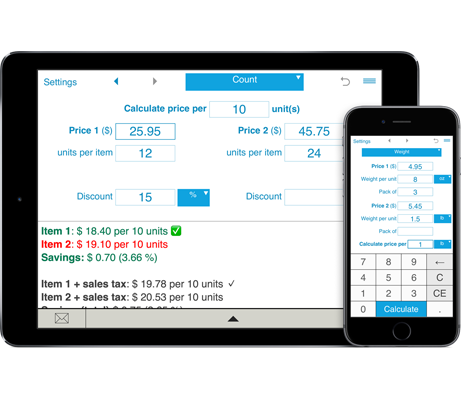# Unit Price Calculator

Not sure you are getting the best deal? Need to compare the same product in different packages at different prices? Is it better to buy 2 4-oz items for \$2.50 or 4 3-oz items for \$1.80?
Unit Price Calculator helps you calculate the price per unit for products of different sizes so you can quickly find the most economic package size and save your time and money.

## Units price comparison

To compare unit prices of items in packages of different sizes, enter the price, quantity and size of comparable items and tap Calculate. Unit Price Calculator supports calculations in US, imperial and metric unit measurement systems.
Supported volume units: fl oz (US), gal (US), fl oz (UK), gal (UK), liter, deciliter, centiliter, milliliter.
Supported weight units: oz, lb, kg, 100 g, gram.

## Compare Units Prices

### How to use the unit price calculator

How to calculate the unit price:

1. Select "Count", "Weight" or "Volume".

2. For Count: enter the number of units per item.

For Weight: enter the weight ("Weight per unit") and the number of items in a pack ("Pack of ..."). For example, for 2 4-oz items, you should enter Weight per unit = 4 oz, Pack of = 2.

For Volume: enter the volume ("Volume per unit") and the number of items in a pack ("Pack of ..."). For example, for 6 16-oz bottles, you should enter Volume per unit = 16 oz, Pack of = 6.

3. Enter the prices of comparable items. The currency can be changed in the Settings window.

4. To calculate the unit price with the Sales Tax, you should pre-define the Sales Tax in the Settings window. In this case, the calculator will show you the price per unit with and without the Sales Tax.

5. With Unit Price Calculator you can compare unit prices with or without discount. Simply enter the discount (percentage or amount) to see which is the better deal, or leave the discount field blank to compare base unit prices of items.

6. Unit Price Calculator lets you calculate the price per pound, ounce, gallon, liter, kilogram, etc. You can calculate the price per any number of units. E.g. you can choose to calculate the price per 5 fl oz, 20 lb, 5 gal, 10 kg, 100 g, 100 ml, 50 l, etc. If you need to show the price per one unit, just leave the "Calculate price per ... unit" field blank or enter "1".

7. Click Calculate.

Unit Price Calculator shows you the price per unit for both items, highlighting the better deal, and showing how much you can save.